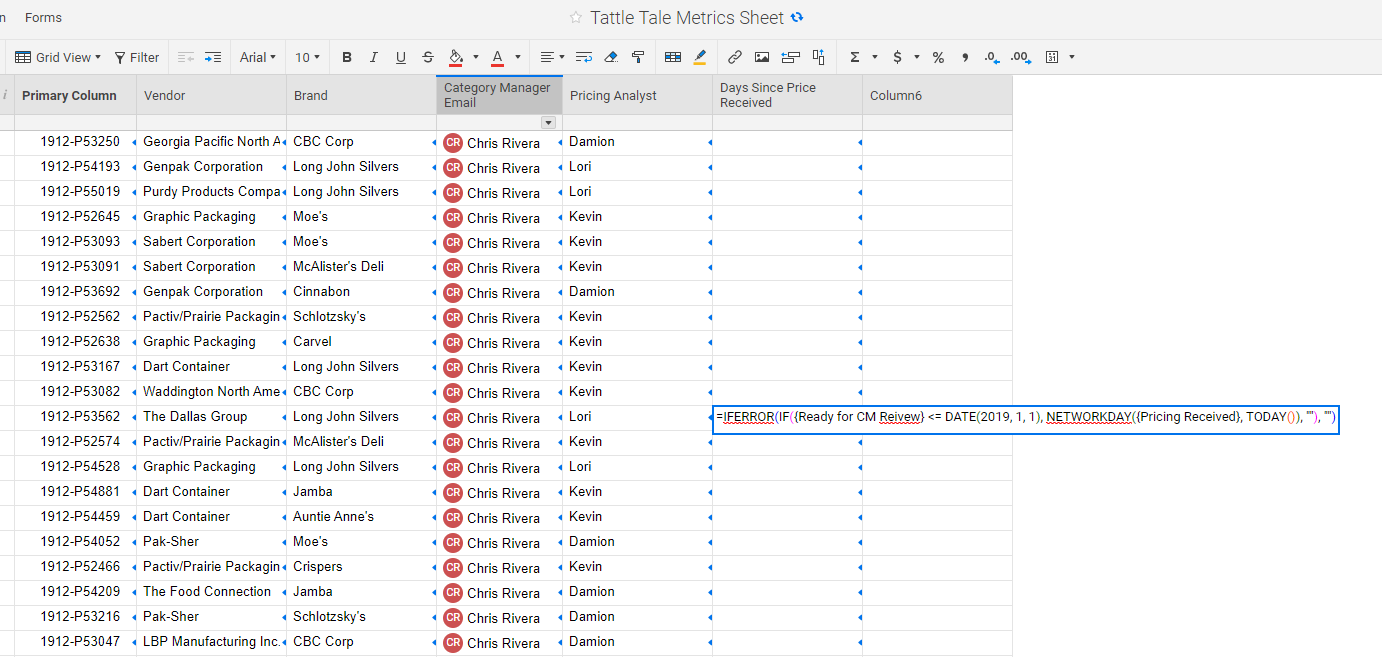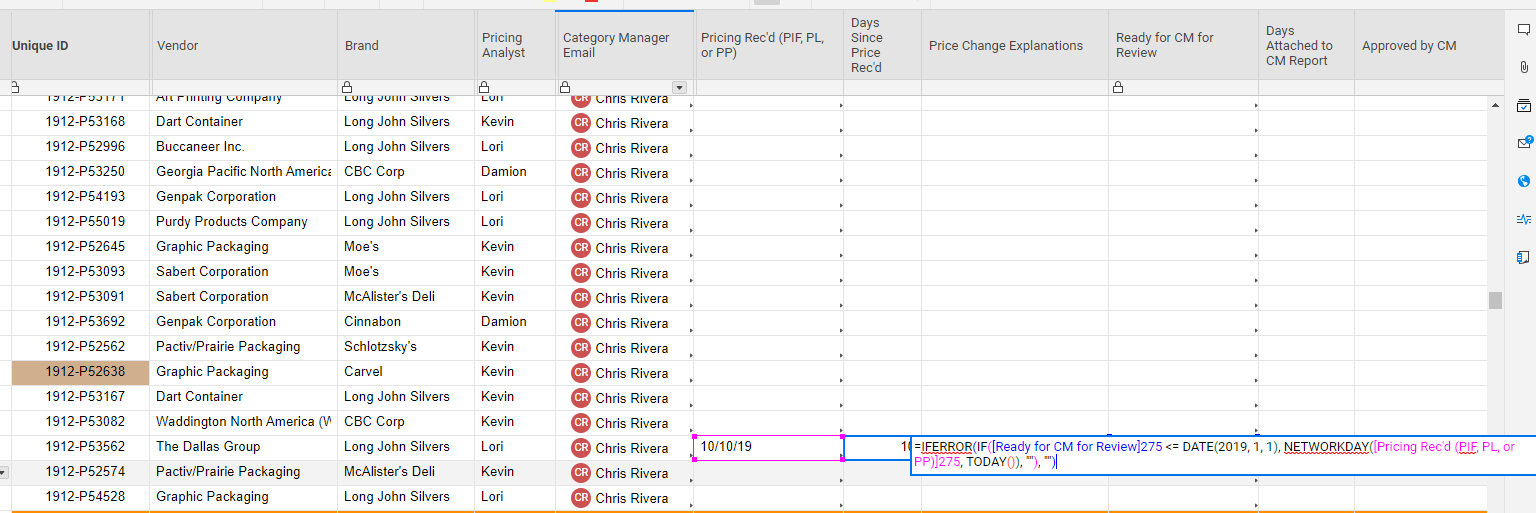# Formulas Referencing Another Sheet

Hi,

am working from a running sheet that currently has formulas but I'm trying to put the formulas into separate sheets so I can run metrics in a slightly different way and try to make the running sheet more efficient. However, whenever I type the exact same formula, with the only difference being referencing another sheet instead of within the same sheet, the formulas don't work.

One formula I'm trying to do is an if statement counting days between two dates. It works in the running sheet where I'm pulling in a column from within the same sheet (and it works) but does not work when I have the same formula in another sheet referencing the same columns in the the running sheet.

I've run across similar instances but this is the best example I have with screenshots.• Hi,

In the cross-sheet formula you are referencing a full column of data in each Sheet Reference (such as {Ready for CM Review}). However, in the original formula you are referencing just two specific cells (for example, [Ready for CM]275, which is only one cell in row 275)

In order to have this cross-sheet formula work for row 275, the sheet reference you used would need to only be for one specific cell (not a whole column). That would mean creating an individual formula for each row, with two distinct cross-sheet references. However, a sheet can only include up to 100 distinct cross-sheet references.

It may be best to keep the formula within that original sheet and then cell-link the data into your new sheet, or create an INDEX(MATCH formula. If your primary column is the same in both sheets, an INDEX(MATCH formula could look for the Primary Column name in both sheets and return the "Days since Price Rec'd" data from the old sheet into this new one.

Alternatively, you could create a report that combines data from your new sheet and original sheet to bring this information together.

## Help Article Resources

Want to practice working with formulas directly in Smartsheet?

Check out the Formula Handbook template!